# HBSE 8th Class Maths Solutions Chapter 4 Practical Geometry Ex 4.3

Haryana State Board HBSE 8th Class Maths Solutions Chapter 4 Practical Geometry Ex 4.3 Textbook Exercise Questions and Answers.

## Haryana Board 8th Class Maths Solutions Chapter 4 Practical Geometry Exercise 4.3

Question 1.
MORE
MO = 6 cm
OR = 4.5 cm
∠M = 60°
∠O = 105°
∠R = 105°
PLAN
PL = 4 cm
LA = 6.5 cm
∠P = 90°
∠A= 110°
∠N = 85°
(iii) Parallel
HEAR
HE = 5 cm
EA = 6 cm
∠R = 85°
PLAN
OK = 7 cm
KA = 5 cm
Solution:
(i) Rough Sketch: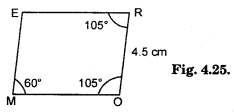Steps of Construction :
(i) Take a line segment MO = 6 cm.
(ii) Construct ∠MOR = 105° and cut off OR = 4.5 cm.
(iii) At R construct ∠ORX = 105°.
(iv) Now, construct ∠OMY = 60°.
(v) Let MY intersect RX at E.
(vi) Thus, MORE is the required quadrilateral.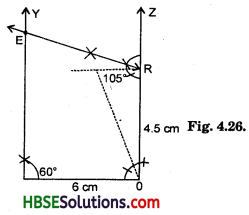(ii) Rough Sketch: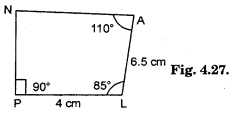Steps of Construction :
(i) Draw a line segment PL = 4 cm.
(ii) Construct ∠P = 90°, ∠L = 85° and cut off ∠A = 6.5 cm in ∠X.
(iii) Construct ∠YPL = 90°
(iv) PY intersect AZ at N.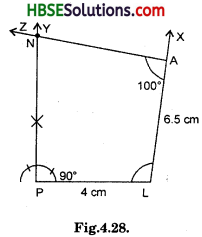(iii) Rough Sketch: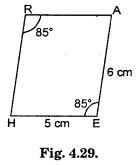Steps of Construction :
(i) Take HE = 5 cm.
(ii) Draw ∠HEA = 85° and cut off EA = 6 cm in EX.
(iii) ∠H = 108° – 85° = 95°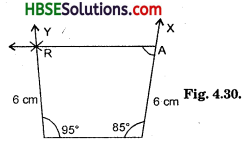(iv) Construct ∠EHY = 95° and cut off HR = 6 cm.
(v) Join HR and RA.
(vi) We have a parallelogram HEAR.(iv) Rough Sketch: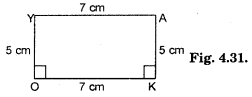We know that each angle of rectangle is 90° and opposite sides are equal.
Steps of Construction :
(i) Draw a line segment OK = 7 cm.
(ii) Construct ∠OKX = 90° and cut off KA = 5 cm.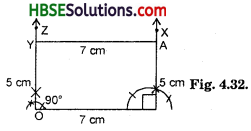(iii) Also, construct ∠KOZ = 90° and cut off OY = 5 cm.
(iv) Join OY and AY.
(v) OKAY is the required rectangle.